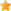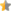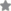Languages and Machines: An Introduction to the Theory of Computer Science, 3/eAuthor(s): Author: Thomas A. Sudkamp ISBN:9788131714751 10 Digit ISBN:8131714756 Price:Rs. 799.00 Pages:672 Imprint:Pearson Education Binding:Paperback Status:Available Ratings:The third edition of Languages and Machines: An Introduction to the Theory of Computer Science provides readers with a mathematically sound presentation of the theory of computer science at a level suitable for junior and senior level computer science majors. The theoretical concepts and associated mathematics are made accessible by a "learn as you go" approach that develops an intuitive understanding of the concepts through numerous examples and illustrations. In this edition the presentation has been enhanced by increasing the number of examples, expanding the selection of topics particularly in the area of computational complexity, and providing a flexible format giving instructors the ability to design their courses that concentrate on specific areas such as automata theory, computability theory, or computational complexity.

Table of Content

1. Foundations
1. Mathematical Preliminaries
2. Languages
2. Grammars, Automata, and Languages
1. Context-Free Grammars
2. Normal Forms for Context-Free Grammars
3. Finite Automata
4. Properties of Regular Languages
5. Pushdown Automata and Context-Free Languages
3. Computability
1. Turing Machines
2. Turing Computable Functions
3. The Chomsky Hierarchy
4. Decision Problems and the Church-Turing Thesis
5. Undecidability
6. Mu-Recursive Functions
4. Computational Complexity
1. Time Complexity
2. P, NP, and Cook's Theorem
3. NP-Complete Problems
5. Deterministic Parsing
1. Parsing: An Introduction
2. LL(k) Grammars
3. LR(k) Grammars
• Appendix I
• Appendix II
• Appendix III
• Appendix IV
• Bibliography
• Subject Index

Salient Features

• Expansion coverage of computational complexity.
• Over 100 new examples and exercises. Examples of programming syntax are given using the BNF description of the programming language Java.
• A new chapter following the definition of NP-completenss and Cook's Theorem presents strategies for demonstrating that a problem is NP-complete.
• Increased coverage of space complexity including Savitch's Theorem and P-space completeness.
• Organized to provide flexibility to design courses that concentrate in specific areas such as automata theory, computability theory, or computational complexity.
• Topics covered with greater emphasis include the use of diagonalization and self-reference in proofs by contradiction, the application of regular expressions in text searching using grep as an example, the CYK parsing algorithm, the motivation for and interpretation of nondeterministic computation, the role of the problem representation in the assessment of computational complexity, and the significance of problem reduction in decidability and undecidability.